BGonline.org Forums

Quantifying recube vig

Posted By: Timothy Chow
Date: Tuesday, 12 January 2010, at 2:35 a.m.

Some time ago, Phil Simborg asked if there is a better way to measure takes (in matches). In this post, I present some preliminary thoughts on this question.

As I understand it, the standard way to decide OTB whether to take (if you really want to try to get it right) goes something like this: First, you determine your "raw take point." This is the percentage of the time you need to win in order to take, assuming no gammons and no further cube action. The raw take point can be derived from a match equity table and depends only on the match score, not on the position. Second, you look at the position and estimate winning chances and gammon chances (assuming it's a position where you can probably ignore backgammons), combining the two after adjusting the gammons by a factor called the "gammon price." The gammon price again depends only on the match score and not the position. Finally, you add in an estimate of the "recube vig," and then compare the result to your raw take point. If wins + adjusted gammons + recube vig exceeds your raw take point, then you take.

The "recube vig" is the part of the above procedure for which, so far, the theoreticians seem to have provided the practical player with the least amount of guidance. However, Daniel Murphy recently drew my attention to the "View Statistics" button in GNU Backgammon. If you ask for a hint and do a rollout, the "View Statistics" button will light up when the rollout is finished, and if you click it, you will get a table displaying, for each possible cube value and outcome (single/gammon/backgammon), how many rollout trials ended that way. You will also see that GNU reports "Cube efficiencies." It is natural to ask, what does all this information mean, and is it of any practical value?

For a concrete example, let me take what I'll call the C-note position:

The score (after 0 games) is: White 4, Blue 8 (match to 13 points)

White39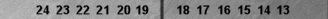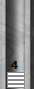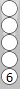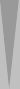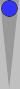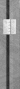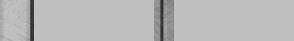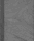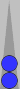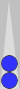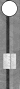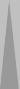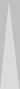Blue83

Position ID: vwcAALRtOwARAA Match ID: UQmgAUAAQAAA

The C-note position is a good example for several reasons: (1) it's a nontrivial position where several world-class players were surprised by the amount of White's recube vig; (2) there are no gammons to complicate the picture; (3) it's an endgame so that we can easily produce enough trials without having to worry about the mathematics of variance reduction.

I did a 15552-game 2-ply/2-ply rollout of the C-note position. "View Statistics" yields the following information, assuming Blue doubles and White takes:

 White wins single Blue wins single 4-cube 780 9037 8-cube 2022 3713

We are also told that there were 5735 games in which White redoubled and Blue took, and 780 games in which White redoubled and Blue passed, yielding what GNU calls a "cube efficiency" of 5735/(5735+780) = 0.8803 (this is the percentage of doubles that are takes).

From all this we can infer that in 9037 of the 15552 trials, Blue won without any further cube action. In all the remaining 15552 � 9037 = 6515 trials, White redoubled; 780 times Blue passed, and 5735 times Blue took. The cube was dead at this point. Blue won 3713 of those 5735 games and White won 2022 of those 5735 games.

Knowing the four numbers in the above table is enough information to compute an estimate of White's (cubeful) match-winning chances. Janowski is not needed; there are four possible outcomes, so we just take a weighted average of the four respective match equities (taken from the g11 MET):

(780/15552)*.5000 + (9037/15552)*0.0541 + (2022/15552)*.8445 + (3713/15552)*0.0000 = 0.166,

which agrees with GNU's variance-reduced estimate of the MWC to 3 decimal places. This is bigger than the 0.1567 MWC that White would have by dropping, so White has a take.

The real question, however, is whether there's any way that we can capture the information in the table in a way that allows our human brains to learn it and use it OTB.

The fact that White wins (780+2022)/15552 = 18% of the time is something we can imagine learning. The fact that all of White's wins involve White turning the cube is also easy to pick up on, if it wasn't already obvious to us. (If we did enough trials then eventually we would get a position where White held on to the cube to the bitter end and then won with lucky doubles on the very last roll, but it's clear this is an extremely rare occurrence.) We might also imagine learning that the "cube efficiency" (as defined by GNU) is 88%, and getting some feel for this number by studying its value in lots of different positions.

We're now close to being able to capture all the information in the table, but we're not quite there yet. We have two pieces of information (18% wins and 88% cube efficiency) but the table contains three pieces of information. (It's three and not four because the four numbers are constrained to sum to 15552, i.e., 100%.) The crucial question, which I don't have an answer to yet, is "what is the best way to represent the third piece of information here?"

There are a few possibilities. One might be, "What is White's redoubling probability (i.e., the percentage of the time that White gets to redouble)?" Another might be, "What percentage of White's redoubles turn out well for White?" Either of these would in principle be enough information to reconstruct the table. (Of course there is still the matter of inventing a simple way to do the calculation in one's head, but I think we should cross that bridge when we get to it.)

I will conclude with some questions for BGOnline readers.

1. Does there already exist a way to approach the issue of recube vig that is better than what I'm pursuing here? I recently read one of Doug Zare's old Gammon Village articles on recube vig, which he defines as "cubeful equity minus cubeless equity." This quantity is (implicitly) reported by the current bots. However, it strikes me as a rather difficult quantity to train oneself to estimate, since so many different factors contribute to it. In contrast, my gut feeling is that cube efficiency (as defined by GNU) and redoubling probability are going to be easier to get a feel for.

2. Assuming for the moment that trying to understand the above table is a good way to understand the position, how would you try to capture the information in a human-digestible way? Does cube efficiency + redoubling probability look promising? If so, then I might take the next step, of figuring out an easy way to do the calculations in one's head, assuming that one has a good estimate of the cube efficiency and the redoubling probability in hand.

Post Response

Subject:
Message: